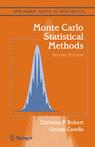## another X’idated questionAn X’idated reader of Monte Carlo Statistical Methods had trouble with our Example 3.13, the very one our academic book reviewer disliked so much as to “diverse [sic] a 2 star”. The issue is with computing the integral$\mathfrak{I}=\int\limits_{-\infty}^{\infty}{\sqrt{\left|\frac{\theta}{1-\theta}\right|}}f(\theta)\text{d}\theta$

when f is the Student’s t(5) distribution density. In our book, we compare a few importance sampling solutions, but it seems someone (the instructor?) suggested to this X’idated reader to use a mixture importance density$0.5\{{{g}_{1}}(\theta )+{{g}_{2}}(\theta )\},$

with${{g}_{1}}(\theta )=\dfrac{1}{\pi }\dfrac{1}{1+{{\theta}^{2}}}$

and${{g}_{2}}(\theta )=\dfrac{1}{4\sqrt{\left|1-\theta\right|}}\quad\text{on}\quad[0,2]$

i.e. with a Cauchy and a power component. The suggested solution in our book is a folded Gamma distribution$g^\prime(\theta)\propto\dfrac{1}{\sqrt{\left|1-\theta\right|}}\exp (-\left|1-\theta\right|).$

The above graph is my final answer to this X’idated reader (after several exchanges in the comments), comparing the three solutions in terms of variability. I used

> m=function(x){
+ sqrt(abs(x/{1-x}))}
> sam1=matrix(rt(10^7,df=5),ncol=100)
> fam1=m(sam1)


for the first box (the matrix is used for computing 100 replicas),

> g=function(x){
+ .5*dcauchy(x)+.125*((x>0)*(x<2))/sqrt(abs(1-x))}
> sam22=1+sample(c(-1,1),5*10^6,rep=TRUE)*runif(5*10^6)^2
> sam21=rcauchy(5*10^6)
> sam2=matrix(sample(c(sam21,sam22)),ncol=100)
> fam2=m(sam2)*dt(sam2,df=5)/g(sam2)


for the second box (with the details that$F(x)=\begin{cases}\dfrac{1}{2}(1-\sqrt{1-x}) &\text{if } 0\le x\le 1\\ \dfrac{1}{2}(1+\sqrt{x-1}) &\text{if } 1\le x\le 2\\\end{cases}$

is the cdf associated with the second component and that it can be easily inverted into 1±u² for simulation,

> sam3=matrix(1+sample(c(-1,1),10^7,
+ rep=TRUE)*rgamma(10^7,.5),ncol=100)
> fam3=m(sam3)*dt(sam3,df=5)/(.5*dgamma(abs(1-sam3),.5))for the third box. The gradual improvement brought by importance sampling is clear there. Which makes me think we should present the comparison that way in the next edition of Monte Carlo Statistical Methods.

### 6 Responses to “another X’idated question”

1.Deinst Says:

I am too lazy to slog through the details, but doesn’t the mixture yield an estimate with finite variance? The Cauchy keeps the tails in order, and the power squashes the singularity. Also on the bottom of page 97, you show that $h_1(x)\frac{f^2(x)}{g(x)}$ is unbounded as opposed to $h_1^2(x)\frac{f^2(x)}{g(x)}$. Is this a typo, or am I missing something? (the expansion on the right looks like a combination of the two)

•xi'an Says:

Thanks! (a) Yes the mixture could work out fine, I am not 100% sure the tail difficulty with the |θ| part is solved though; (b) p.97, this is is indeed a typo!

•Deinst Says:

a) In the tails h_1^2 is approximately 1, and certainly bounded. f/g is \$(1+x^2)/(1+x^2/5)^3 which goes to 0, and is again, certainly bounded. So the integral of h^2f^2/g in the tails is bounded by some constant times the integral of f over the tails, which exists.

c) Shouldn’t the code for sam3 be something like

sam3 = matrix(1 + sample(c(-1, 1), 10^7, rep=TRUE) * rgamma(10^7, 0.5), ncol=100)

else you have 5 times as many sample points in the third group. (the mixture comes out slightly better when all of the samples are the same size.)

•xi'an Says:

Thank you, the sam3 error is indeed blatant! A result of a poor cut&paste… I have corrected it.

•xi'an Says:

And I have just corrected the graph to account for the same number of simulations. The mixture proposal does look better, indeed.

•deinst Says:

An even better estimator is to use a mixture of the power and the underlying t distribution. This is motivated by the theory that one wants |h|f/g to be almost constant. This seems to have about half the variance of the Cauchy-power estimator, at the cost of a bit more work. Sampling from the Cauchy distribution is straightforward, sampling from the t-distribution, less so, but on my machine I get half the variance from about 1.5 times the work, so I’ll call it a win.

This site uses Akismet to reduce spam. Learn how your comment data is processed.